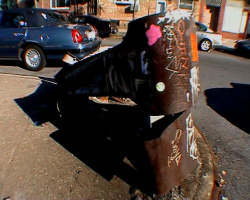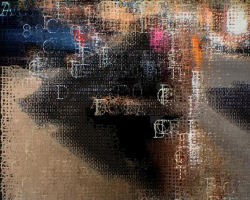# Python

### Code from the pixelstudy Bike & Planke, 2004

<>

2 scripts for the distinct display of pixelinfos within a video
The result can be seen in the section Multimedia - 'Bike & Planke'.

#### Here's the 1. script for displaying pixels as #hex values

the size of the displaxed #hex letters is due to the luminance:#!/usr/bin/python2.3 # this code is written in 2004 by sesselastronaut@gmail.com & is very basic # but it's working... # check the output on http://hasa-labs.org/orbit_en/pixelstudy.php # it processes a series of singleframes starting with the regular image # fading into the display of the #hex values of the displayed pixels at the # middle of the series back into the regular image - though i've tried to # comment it a bit i might be the only person who understands what's going on, # so please do not hesitate to contact me if you've troubles or questions. #import import os, sys import Image import ImageDraw import ImageFont #get files from this folder filepath = "/path_to_single_frames_numbered/" #save files here savepath = "/path_where_to_save_the_processed_frames/" #number of startframe start = 10 #number of endframe end = 696 suffix = ".jpg" #middle of the 'movie' - starting to fade back from displaying hex-values to the regular image semiseq = ((end - start)/2) print "there are", 2*semiseq, "frames to decode" def hexdraw(): for i in range(start, end): # %s - string, %08i - integer mit 8 führenden nullen, %s - string filename = "%s%06i%s" % (filepath, i, suffix) print "File: %s"% file, "Frame=", i ##image openeer img = Image.open (filename) print 'image=', img.format, img.size, img.mode canvas1 = Image.new ( "RGBA", img.size , (0,0,0,0)) print 'canvas=', canvas1.format, canvas1.size, canvas1.mode xmax = int(img.size) ymax = int(img.size) print xmax, ymax x = 0 y = 0 #loop n draw while x < (xmax): #width/x pixel while y < ymax: #print 'ypiksel=', y, 'xpixel =', x res = 10 rgb = img.getpixel ((x, y)) lum = int(0.299*rgb + 0.587*rgb + 0.114*rgb)+72 hex = "#%02X%02X%02X" % rgb #path to fonts you would like to be used #i used /usr/share/fonts/truetype/freefont/FreeMono.ttf font1 = ImageFont.truetype('/Path_to_the_font/font.ttf', lum) font2 = ImageFont.truetype('/Path_to_the_font/font.ttf', lum/2) font3 = ImageFont.truetype('/Path_to_the_font/font.ttf', lum/3) font4 = ImageFont.truetype('/Path_to_the_font/font.ttf', lum/4) draw = ImageDraw.Draw (canvas1) draw.text ((x-1, y-12) , hex[1:], font=font4, fill=rgb) y = y+res y = 0 x = x+res im = img.convert("RGBA") mix = float(1)/semiseq middle = start + semiseq if i < middle: f = i-start mixed_canv = Image.blend (im, canvas1, f*mix) else: f = end-i mixed_canv = Image.blend (im,canvas1, f*mix) #f = i-(2*semiseq-start) print "there are ",end-i,"frames left" #mixed_canv = Image.blend (im, canvas1, 0.1) #mixed_canv.show() mixed_canv.save(("%s%06i%s" % (savepath, i , suffix)),"JPEG") hexdraw()

#### & here the 2. script which

1. relocates the #hex-values of pixels in a coordinate system - the rgb value matches the xyz coordinates
2. represents pixels as colored arcs - radius due to luminanz - deformation due to differenz of neighbourpixels.

#!/usr/bin/python2.3 # this code is written in 2004 by sesselastronaut@gmail.com & is very basic # but it's working... # check the output on http://hasa-labs.org/orbit_en/pixelstudy.php # it processes a series of singleframes: # 1. it draws the #hex-values of the pixels relocated in a coordinate system - # rgb value = xyz # 2. it draws pixels represented as colored arcs - radius due to luminanz & # deformation due to differenz of neighbourpixels. though i've tried to # comment it a bit i might be the only person who understands what's going on, # so please do not hesitate to contact me if you've troubles or questions. #import import os, sys, math import Image import ImageDraw import ImageFont #get files from this folder filepath = "/path_to_single_frames_numbered/" #save files displayed in coordinate system here savepath = "/path_where_to_save_the_processed_rgb_frames/" #save files displayed as arcs here savepath = "/path_where_to_save_the_processed_arc_frames/" #number of startframe start = 10 #number of endframe end = 696 suffix = ".jpg" #middle of the 'movie' - starting to fade back from displaying hex-values to the regular image semiseq = ((end - start)/2) print "das ding brauch", semiseq, "*2 frames" #converts a point(x,y,z) in 3-dim for 2-dim display(x,y) def z_2dim(x,y,z): degrees = -30 angle = degrees * 2 * math.pi /360.0 x = x+math.cos(angle)*z y = y+math.sin(angle)*z return x,y def hexdraw(): for i in range(start, end): # %s - string, %08i - integer mit 8 führenden nullen, %s - string filename = "%s%06i%s" % (filepath, i, suffix) print "File: %s"% file, "Frame=", i ##image openeer img = Image.open (filename) print 'image=', img.format, img.size, img.mode #arc canvas arc_canvas = Image.new ( "RGB", (800,240) , (185,200,230) ) #rgb canvas rgb_canvas = Image.new ( "RGB", (800,720) , (185,200,230) ) print 'canvas=', arc_canvas.format, arc_canvas.size, arc_canvas.mode xmax = int(img.size) ymax = int(img.size) print xmax, ymax x = 0 y = 0 #offset osx = 730 osy = 150 lum2 = 0 v = -250 #loop n draw while x < (xmax): #width/x pixel while y < ymax: #print 'ypiksel=', y, 'xpixel =', x res = 15 rgb = img.getpixel ((x, y)) lum = int(0.299*rgb + 0.587*rgb + 0.114*rgb) hex = "#%02X%02X%02X" % rgb #path to fonts you would like to be used #i used /usr/share/fonts/truetype/freefont/FreeMono.ttf font = ImageFont.truetype('/Path_to_the_font/font.ttf', lum/4) draw2 = ImageDraw.Draw (arc_canvas) draw = ImageDraw.Draw (rgb_canvas) draw = ImageDraw.Draw (arc_canvas) xy = z_2dim(rgb,rgb,rgb) #print "xyz2xy=", xy #luminance diffrence between this and pixel before lum_diff = lum-lum2 draw2.text ((xy+10,xy+10), hex[:6], font=font, fill=rgb) draw2.line (((10,10),(xy+10,xy+10)), fill=rgb) x1=(osx+x-((xy+lum-lum_diff)/2))/2 y1=(osy+y-((xy+lum)/2))/2 x2=(osx+x+((xy+lum-lum_diff)/2))/2 y2=(osy+y+((xy+lum)/2))/2 draw.arc((x1,y1,x2,y2), 0, 360, fill=rgb) lum2 = lum y = y+res y = 0 x = x+res #im = img.convert("RGBA") arc_canvas.save(("%s%06i%s" % (savepath, i , suffix)),"JPEG") rgb_canvas.save(("%s%06i%s" % (savepath2, i , suffix)),"JPEG") #canvas1.show () hexdraw()

#### These are the steps to prepare & convert the singleframes for the videos:

Video Stream de/encoding is done with mplayer/mencoder:http://www.mplayerhq.hu
1. export movie to single jpegs:
mplayer -vop scale=720:576,eq=-20,hue=0.0:-2.1 -nosound -vo jpeg -jpeg optimize=6:quality=6 nameofmovie.mov
2. cut out some frames(processing 25fps take2much time in my machine):
rm -f *~.jpg ->13fps
3. rename frames in a row with the help of this little bash script:

#! /bin/bash n=1 ## initialize first file number z=0000 ## a string for padding with zeroes for file in *.jpg ## for each file ending with .jpg do f=\$z\$n ## put the zeroes ahead of the number newfile=\${f: -6}.jpg ## use the last 4 characters of \$f mv "\$file" "\$newfile" ## move 'em out n=\$(( \$n + 1 )) ## add one to \$n done

3. execute the python scripts from above
4. recode single .jpg in a folder to stream: mencoder "mf://*.jpg" -mf fps=25 -o output.avi -ovc lavc -lavcopts vcodec=mpeg4
5. eat icecream